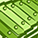# QlikView App Dev

Discussion Board for collaboration related to QlikView App Development.

Announcements
Skip the ticket, Chat with Qlik Support instead for instant assistance.
cancel
Showing results for
Did you mean:Partner - Contributor

## cumulative sum in the graph

Using this formula, I can obtain commutative sum for each category:

=Rangesum( above(  sum( {\$<Month=>} Amount),0,RowNo()))

The problem is, when I use the same formula in a chart, the result obtained is not the same.

In the screenshot below, Graph is the clone of "Table 1", however it doesn't reflect the same values:My desired graph is the one with the same value as the Table 1 's "Cumulative sum" column for each category.

The problem is RowNo() function has different behavior when used inside the graph rather than as a script.

I would like to apply the solution without using the "Full accumulation" option.Partner - Contributor

use this in frontend for your desire result-

aggr(RangeSum(Above((Sum(Amount)),0,RowNo())),Year,Month)Community Browser